Used in everything from microcontrollers to operating systems, C is a popular programming language among developers because of its flexibility and versatility. This book helps you get hands-on with various tasks, covering the fundamental as well as complex C programming concepts that are essential for making real-life applications.

You’ll start with recipes for arrays, strings, user-defined functions, and pre-processing directives. Once you’re familiar with the basic features, you’ll gradually move on to learning pointers, file handling, concurrency, networking, and inter-process communication (IPC). The book then illustrates how to carry out searching and arrange data using different sorting techniques, before demonstrating the implementation of data structures such as stacks and queues. Later, you’ll learn interesting programming features such as using graphics for drawing and animation, and the application of general-purpose utilities. Finally, the book will take you through advanced concepts such as low-level programming, embedded software, IoT, and security in coding, as well as techniques for improving code performance.

By the end of this book, you'll have a clear understanding of C programming, and have the skills you need to develop robust apps.

Publication date:
February 2020
Publisher
Packt
Pages
616
ISBN
9781838641108

# Working with Arrays

Arrays are an important construct of any programming language. To keep data of a similar type together, we need arrays. Arrays are heavily used in applications where elements have to be accessed at random. Arrays are also a prime choice when you need to sort elements, look for desired data in a collection, and find common or unique data between two sets. Arrays are assigned contiguous memory locations and are a very popular structure for sorting and searching data collections because any element of an array can be accessed by simply specifying its subscript or index location. This chapter will cover recipes that include operations commonly applied to arrays.

In this chapter, we will learn how to make the following recipes using arrays:

• Inserting an element into a one-dimensional array
• Multiplying two matrices
• Finding the common elements in two arrays
• Finding the difference between two sets or arrays
• Finding the unique elements in an array
• Finding whether a matrix is sparse
• Merging two sorted arrays into one

Let's begin with the first recipe!

# Inserting an element in an array

In this recipe, we will learn how to insert an element in-between an array. You can define the length of the array and also specify the location where you want the new value to be inserted. The program will display the array after the value has been inserted.

# How to do it…

1. Let's assume that there is an array, p, with five elements, as follows: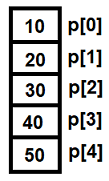Figure 1.1

Now, suppose you want to enter a value, say 99, at the third position. We will write a C program that will give the following output: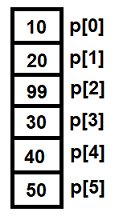Figure 1.2

Here are the steps to follow to insert an element in an array:

1. Define a macro called max and initialize it to a value of 100:
`#define max 100`
1. Define an array p of size max elements:
`int p[max]`
1. Enter the length of the array when prompted. The length you enter will be assigned to a variable n:
`printf("Enter length of array:");scanf("%d",&n);`
1. A for loop will be executed prompting you to enter the elements of the array:
`for(i=0;i<=n-1;i++ )    scanf("%d",&p[i]);`
1. Specify the position in the array where the new value has to be inserted:
`printf("\nEnter position where to insert:");scanf("%d",&k);`
1. Because the arrays in C are zero-based, the position you enter is decremented by 1:
`k--;`
1. To create space for the new element at the specified index location, all the elements are shifted one position down:
`for(j=n-1;j>=k;j--)    p[j+1]=p[j];`
1. Enter the new value which will be inserted at the vacated index location:
`printf("\nEnter the value to insert:");scanf("%d",&p[k]);`

Here is the insertintoarray.c program for inserting an element in between an array:

`#include<stdio.h>#define max 100void main(){    int p[max], n,i,k,j;    printf("Enter length of array:");    scanf("%d",&n);    printf("Enter %d elements of array\n",n);    for(i=0;i<=n-1;i++ )        scanf("%d",&p[i]);    printf("\nThe array is:\n");    for(i = 0;i<=n-1;i++)        printf("%d\n",p[i]);    printf("\nEnter position where to insert:");    scanf("%d",&k);    k--;/*The position is always one value higher than the subscript, so it is decremented by one*/                 for(j=n-1;j>=k;j--)        p[j+1]=p[j];    /* Shifting all the elements of the array one position down from the location of insertion */    printf("\nEnter the value to insert:");    scanf("%d",&p[k]);    printf("\nArray after insertion of element: \n");    for(i=0;i<=n;i++)        printf("%d\n",p[i]);}`

Now, let's go behind the scenes to understand the code better.

# How it works...

Because we want to specify the length of the array, we will first define a macro called max and initialize it to a value of 100. I have defined the value of max as 100 because I assume that I will not need to enter more than 100 values in an array, but it can be any value as desired. An array, p, is defined of size max elements. You will be prompted to specify the length of the array. Let's specify the length of the array as 5. We will assign the value 5 to the variable n. Using a for loop, you will be asked to enter the elements of the array.

Let's say you enter the values in the array, as shown in Figure 1.1 given earlier: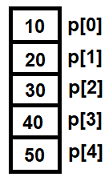In the preceding diagram, the numbers, 0, 1, 2, and so on are known as index or subscript and are used for assigning and retrieving values from an array. Next, you will be asked to specify the position in the array where the new value has to be inserted. Suppose, you enter 3, which is assigned to the variable k. This means that you want to insert a new value at location 3 in the array.

Because the arrays in C are zero-based, position 3 means that you want to insert a new value at index location 2, which is p. Hence, the position entered in k is decremented by 1.

To create space for the new element at index location p, all the elements are shifted one position down. This means that the element at p is moved to index location p, the one at p is moved to p, and the element at p is moved to p, as follows: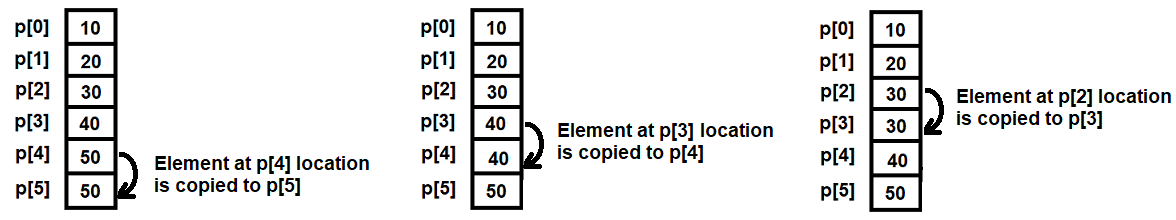Figure 1.3

Once the element from the target index location is safely copied to the next location, you will be asked to enter the new value. Suppose you enter the new value as 99; that value will be inserted at index location p, as shown in Figure 1.2, given earlier: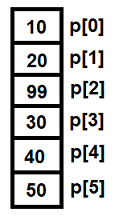Let’s use GCC to compile the insertintoarray.c program, as shown in this statement:

`D:\CBook>gcc insertintoarray.c -o insertintoarray`

Now, let’s run the generated executable file, insertintoarray.exe, to see the program output:

`D:\CBook>./insertintoarrayEnter length of array:5Enter 5 elements of array1020304050The array is:1020304050Enter target position to insert:3Enter the value to insert:99Array after insertion of element:102099304050`

Voilà! We've successfully inserted an element in an array.

# There's more...

What if we want to delete an element from an array? The procedure is simply the reverse; in other words, all the elements from the bottom of the array will be copied one place up to replace the element that was deleted.

Let's assume array p has the following five elements (Figure 1.1):Suppose, we want to delete the third element, in other words, the one at p, from this array. To do so, the element at p will be copied to p, the element at p will be copied to p, and the last element, which here is at p, will stay as it is: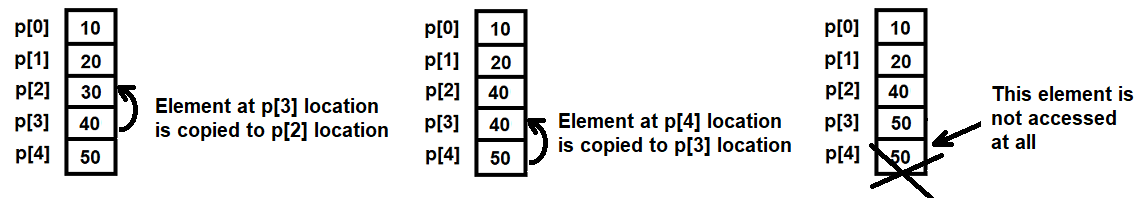Figure 1.4

The deletefromarray.c program for deleting the array is as follows:

`#include<stdio.h>void main(){    int p,i,n,a;    printf("Enter the length of the array: ");    scanf("%d",&n);    printf("Enter %d elements of the array \n",n);    for(i=0;i<=n-1;i++)        scanf("%d",&p[i]);    printf("\nThe array is:\n");\    for(i=0;i<=n-1;i++)        printf("%d\n",p[i]);    printf("Enter the position/location to delete: ");    scanf("%d",&a);    a--;    for(i=a;i<=n-2;i++)    {        p[i]=p[i+1];        /* All values from the bottom of the array are shifted up till         the location of the element to be deleted */    }    p[n-1]=0;    /* The vacant position created at the bottom of the array is set to     0 */    printf("Array after deleting the element is\n");    for(i=0;i<= n-2;i++)        printf("%d\n",p[i]);}`

Now, let's move on to the next recipe!

# Multiplying two matrices

A prerequisite for multiplying two matrices is that the number of columns in the first matrix must be equal to the number of rows in the second matrix.

# How to do it…

1. Create two matrices of orders 2 x 3 and 3 x 4 each.
2. Before we make the matrix multiplication program, we need to understand how matrix multiplication is performed manually. To do so, let's assume that the two matrices to be multiplied have the following elements: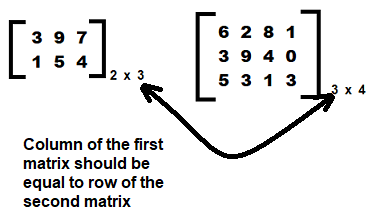Figure 1.5
1. The resultant matrix will be of the order 2 x 4, that is, the resultant matrix will have the same number of rows as the first matrix and the same number of columns as the second matrix: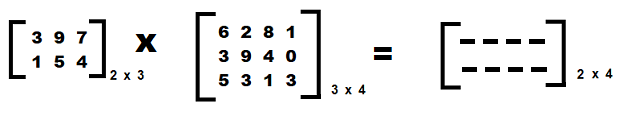Figure 1.6

Essentially, the resultant matrix of the order 2 x 4 will have the following elements: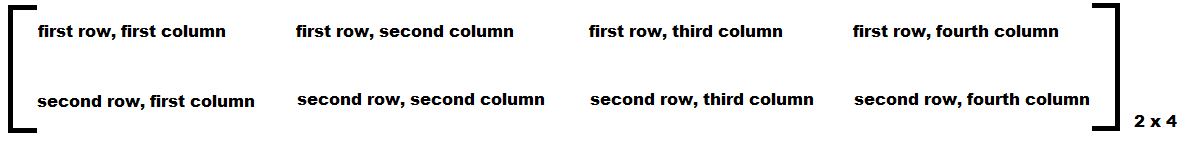Figure 1.7
1. The element first row, first column in the resultant matrix is computed using the following formula:

SUM(first element of the first row of the first matrix × first element of the first column of the second matrix), (second element of the first row... × second element of the first column...), (and so on...)

For example, let's assume the elements of the two matrices are as shown in Figure 1.5.  The elements in the first row and the first column of the resultant matrix will be computed as follows: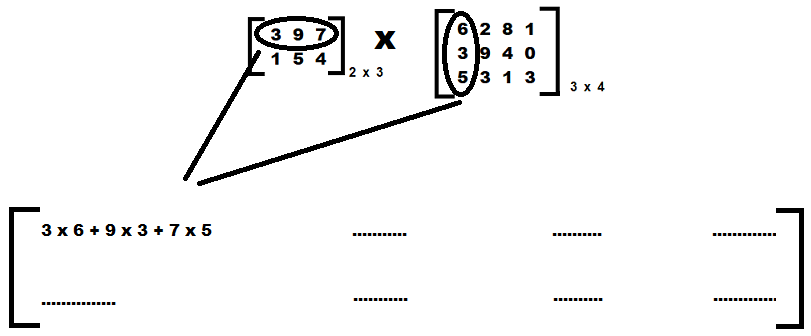Figure 1.8
1. Hence, the element in first row, first column in the resultant matrix will be as follows:

(3×6)+(9×3)+(7×5)
=18 + 27 + 35
=80

Figure 1.9 explains how the rest of the elements are computed in the resultant matrix: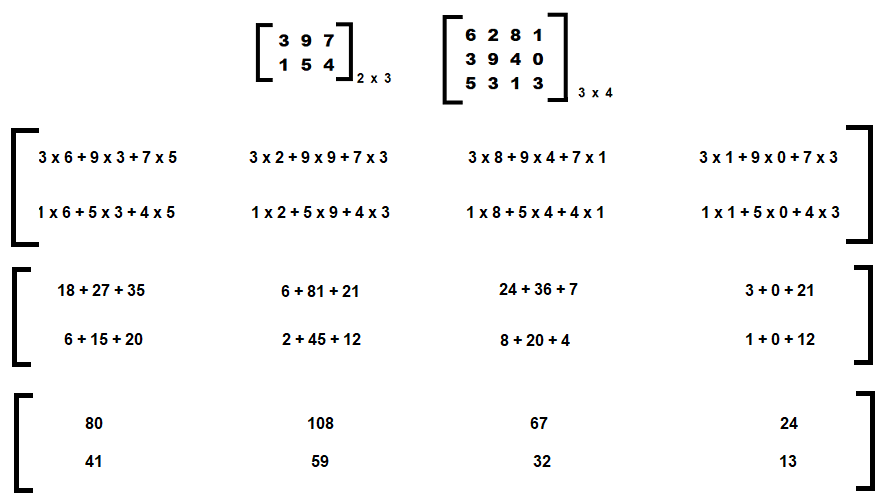Figure 1.9

The matrixmulti.c program for multiplying the two matrices is as follows:

`#include  <stdio.h>int main(){  int matA, matB, matR;  int i,j,k;  printf("Enter elements of the first matrix of order 2 x 3 \n");  for(i=0;i<2;i++)  {    for(j=0;j<3;j++)    {      scanf("%d",&matA[i][j]);    }  }  printf("Enter elements of the second matrix of order 3 x 4 \n");  for(i=0;i<3;i++)  {    for(j=0;j<4;j++)    {      scanf("%d",&matB[i][j]);    }  }  for(i=0;i<2;i++)  {    for(j=0;j<4;j++)    {      matR[i][j]=0;      for(k=0;k<3;k++)      {        matR[i][j]=matR[i][j]+matA[i][k]*matB[k][j];      }    }  }  printf("\nFirst Matrix is \n");  for(i=0;i<2;i++)  {    for(j=0;j<3;j++)    {      printf("%d\t",matA[i][j]);    }    printf("\n");  }  printf("\nSecond Matrix is \n");  for(i=0;i<3;i++)  {    for(j=0;j<4;j++)    {      printf("%d\t",matB[i][j]);    }    printf("\n");  }  printf("\nMatrix multiplication is \n");  for(i=0;i<2;i++)  {    for(j=0;j<4;j++)    {      printf("%d\t",matR[i][j]);    }    printf("\n");  }  return 0;}`

Now, let's go behind the scenes to understand the code better.

# How it works...

The two matrices are defined matA and matB of the orders 2 x 3 and 3 x 4, respectively, using the following statement:

`int matA, matB`

You will be asked to enter the elements of the two matrices using the nested for loops. The elements in the matrix are entered in row-major order, in other words, all the elements of the first row are entered first, followed by all the elements of the second row, and so on.

In the nested loops, for i and for j, the outer loop, for i, represents the row and the inner loop, and for j represents the column.

While entering the elements of matrices matA and matB, the values entered in the two matrices will be assigned to the respective index locations of the two-dimensional arrays as follows: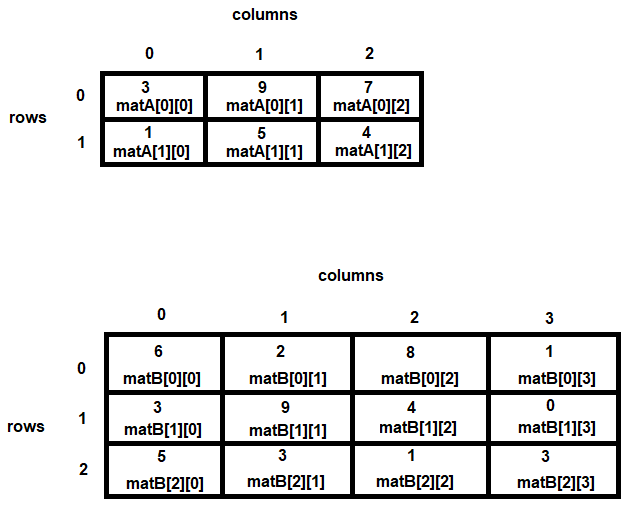Figure 1.10

The nested loops that actually compute the matrix multiplication are as follows:

`  for(i=0;i<2;i++)  {    for(j=0;j<4;j++)    {      matR[i][j]=0;      for(k=0;k<3;k++)      {        matR[i][j]=matR[i][j]+matA[i][k]*matB[k][j];      }    }  }`

The variable i represents the row of the resultant matrix, j represents the column of the resultant matrix, and k represents the common factor. The common factor here means the column of the first matrix and the row of the second matrix.

Recall that the prerequisite for matrix multiplication is that the column of the first matrix should have the same number of rows as the second matrix. Because the respective elements have to be added after multiplication, the element has to be initialized to 0 before addition.

The following statement initializes the elements of the resultant matrix:

`      matR[i][j]=0;`

The for k loop inside the nested loops helps in selecting the elements in the rows of the first matrix and multiplying them by elements of the column of the second matrix:

`matR[i][j]=matR[i][j]+matA[i][k]*matB[k][j];`

Let's use GCC to compile the matrixmulti.c program as follows:

`D:\CBook>gcc matrixmulti.c -o matrixmulti`

Let's run the generated executable file, matrixmulti.exe, to see the output of the program:

`D:\CBook\Chapters\1Arrays>./matrixmultiEnter elements of the first matrix of order 2 x 3397154Enter elements of the second matrix of order 3 x 46 2 8 13 9 4 05 3 1 3First Matrix is3 9 7 1 5 4Second Matrix is6 2 8 13 9 4 05 3 1 3Matrix multiplication is80 108 67 2441 59 32 13`

Voilà! We've successfully multiplied two matrices.

# There’s more…

One thing that you might notice while entering the elements of the matrix is that there are two ways of doing it.

1. The first method is that you press Enter after inputting each element as follows:
`397154`

The values will be automatically assigned to the matrix in row-major order, in other words, 3 will be assigned to matA, 9 will be assigned to matA, and so on.

1. The second method of entering elements in the matrix is as follows:
`6 2 8 13 9 4 05 3 1 3`

Here, 6 will be assigned to matB, 2 will be assigned to matB, and so on.

Now, let's move on to the next recipe!

# Finding the common elements in two arrays

Finding the common elements in two arrays is akin to finding the intersection of two sets. Let's learn how to do it.

# How to do it…

1. Define two arrays of a certain size and assign elements of your choice to both the arrays. Let's assume that we created two arrays called p and q, both of size four elements: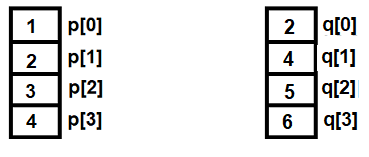Figure 1.11
1. Define one more array. Let's call it array r, to be used for storing the elements that are common between the two arrays.
2. If an element in array p exists in the array q, it is added to array r. For instance, if the element at the first location in array p, which is at p, does not appear in array q, it is discarded, and the next element, at p, is picked up for comparison.
1. And if the element at p is found anywhere in array q, it is added to array r, as follows: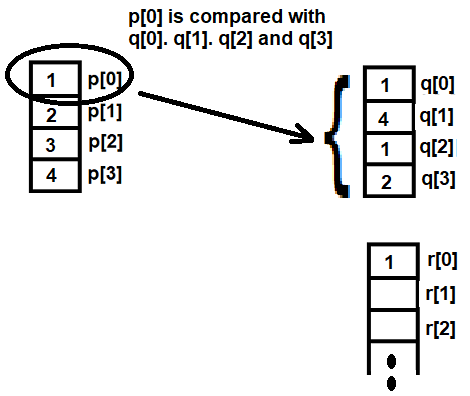Figure 1.12
1. This procedure is repeated with other elements of array q. That is, p is compared with q, q, q, and q. If p is not found in array q, then before inserting it straightaway into array r, it is compared with the existing elements of array r to avoid repetitive elements.
2. Because the element at p appears in array q and is not already present in array r, it is added to array r as follows: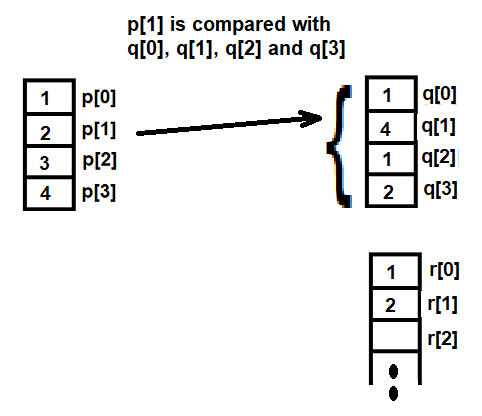Figure 1.13

The commoninarray.c program for establishing common elements among the two arrays is as follows:

`#include<stdio.h>#define max 100int ifexists(int z[], int u, int v){    int i;    if (u==0) return 0;    for (i=0; i<=u;i++)        if (z[i]==v) return (1);    return (0);}void main(){    int p[max], q[max], r[max];    int m,n;    int i,j,k;    k=0;    printf("Enter the length of the first array:");    scanf("%d",&m);    printf("Enter %d elements of the first array\n",m);    for(i=0;i<m;i++ )        scanf("%d",&p[i]);    printf("\nEnter the length of the second array:");    scanf("%d",&n);    printf("Enter %d elements of the second array\n",n);    for(i=0;i<n;i++ )        scanf("%d",&q[i]);    k=0;    for (i=0;i<m;i++)    {        for (j=0;j<n;j++)        {           if (p[i]==q[j])           {               if(!ifexists(r,k,p[i]))               {                   r[k]=p[i];                   k++;               }            }        }    }    if(k>0)    {        printf("\nThe common elements in the two arrays are:\n");        for(i = 0;i<k;i++)            printf("%d\n",r[i]);    }    else        printf("There are no common elements in the two arrays\n");}`

Now, let's go behind the scenes to understand the code better.

# How it works...

A macro, max, is defined of size 100. A function, ifexists(), is defined that simply returns true (1) or false (0). The function returns true if the supplied value exists in the specified array, and false if it doesn't.

Two arrays are defined, called p and q, of size max (in other words, 100 elements). You will be prompted to specify the length of the array, p, and then asked to enter the elements in that array. After that, you will be asked to specify the length of array q, followed by entering the elements in array q.

Thereafter, pthe first element in array p , is picked up, and by using the for loop, p is compared with all the elements of array q. If p is found in array q, then p is added to the resulting array, r.

After a comparison of p, the second element in array p, p, is picked up and compared with all the elements of array q. The procedure is repeated until all the elements of array p are compared with all the elements of array q.

If any elements of array p are found in array q, then before adding that element to the resulting array, r, it is run through the ifexists() function to ensure that the element does not already exist in array r. This is because we don't want repetitive elements in array r.

Finally, all the elements in array r, which are the common elements of the two arrays, are displayed on the screen.

Let's use GCC to compile the commoninarray.c program as follows:

`D:\CBook>gcc commoninarray.c -o commoninarray`

Now, let's run the generated executable file, commoninarray.exe, to see the output of the program:

`D:\CBook>./commoninarrayEnter the length of the first array:5Enter 5 elements in the first array12345Enter the length of the second array:4Enter 4 elements in the second array7890There are no common elements in the two arrays`

Because there were no common elements between the two arrays entered previously, we can't quite say that we've truly tested the program. Let's run the program again, and this time, we will enter the array elements such that they have something in common.

`D:\CBook>./commoninarrayEnter the length of the first array:4Enter 4 elements in the first array1234Enter the length of the second array:4Enter 4 elements in the second array1412The common elements in the two arrays are:124`

Voilà! We've successfully identified the common elements between two arrays.

# Finding the difference between two sets or arrays

When we talk about the difference between two sets or arrays, we are referring to all the elements of the first array that don't appear in the second array. In essence, all the elements in the first array that are not common to the second array are referred to as the difference between the two sets. The difference in sets p and q, for example, will be denoted by p – q.

If array p, for example, has the elements {1, 2, 3, 4}, and array q has the elements {2, 4, 5, 6}, then the difference between the two arrays, p - q, will be  {1,3}.  Let's find out how this is done.

# How to do it…

1. Define two arrays, say p and q, of a certain size and assign elements of your choice to both the arrays.
2. Define one more array, say r, to be used for storing the elements that represent the difference between the two arrays.
3. Pick one element from array p and compare it with all the elements of the array q.
4. If the element of array p exists in array q, discard that element and pick up the next element of array p and repeat from step 3.
5. If the element of array p does not exist in array q, add that element in array r. Before adding that element to array r, ensure that it does not already exist in array r.
6. Repeat steps 3 to 5 until all the elements of array p are compared.
7. Display all the elements in array r, as these are the elements that represent the difference between arrays p and q.

The differencearray.c program to establish the difference between two arrays is as follows:

`#include<stdio.h>#define max 100int ifexists(int z[], int u, int v){    int i;    if (u==0) return 0;    for (i=0; i<=u;i++)        if (z[i]==v) return (1);    return (0);}void main(){    int p[max], q[max], r[max];    int m,n;    int i,j,k;    printf("Enter length of first array:");    scanf("%d",&m);    printf("Enter %d elements of first array\n",m);    for(i=0;i<m;i++ )        scanf("%d",&p[i]);    printf("\nEnter length of second array:");    scanf("%d",&n);    printf("Enter %d elements of second array\n",n);    for(i=0;i<n;i++ )                                                                                    scanf("%d",&q[i]);    k=0;    for (i=0;i<m;i++)                   {                                        for (j=0;j<n;j++)                                        {            if (p[i]==q[j])            {                                                                                                                                    break;                                                               }        }        if(j==n)        {            if(!ifexists(r,k,p[i]))                                                           {                r[k]=p[i];                k++;            }        }    }    printf("\nThe difference of the two array is:\n");    for(i = 0;i<k;i++)        printf("%d\n",r[i]);}`

Now, let's go behind the scenes to understand the code better.

# How it works...

We defined two arrays called p and q. We don't want to fix the length of these arrays, so we should define a macro called max of value 100 and set the two arrays, p and q, to the size of max.

Thereafter, you will be prompted to specify the size of the first array and enter the elements in the first array, p. Similarly, you will be asked to specify the length of the second array, q, followed by entering the elements in the second array.

Let's assume you have specified the length of both arrays as 4 and have entered the following elements:Figure 1.14

We need to pick up one element at a time from the first array and compare it with all the elements of the second array. If an element in array p does not appear in array q, it will be assigned to the third array we created, array r.

Array r will be used for storing the elements that define the difference between two arrays. As shown in Figure 1.15, the first element of array p, in other words, at p, is compared with all the elements of array q, in other words, with q, q, q, and q.

Because the element at p, which is 1, does not appear in array q, it will be added to the array r, indicating the first element representing the difference between the two arrays: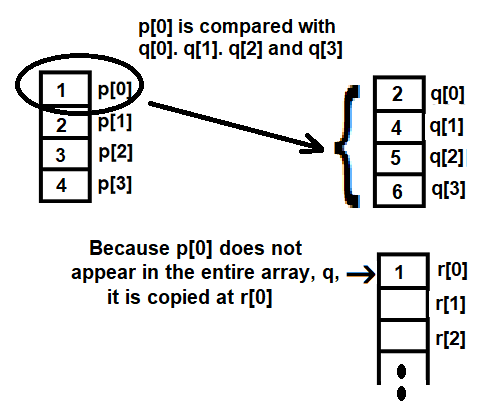Figure 1.15

Because the element at p, which is 2, appears in array q, it is discarded, and the next element in array p, in other wordsp, is picked up and compared with all the elements in array q.

As the element at p does not appear in array q, it is added to array r at the next available location, which is r (see Figure 1.16 as follows):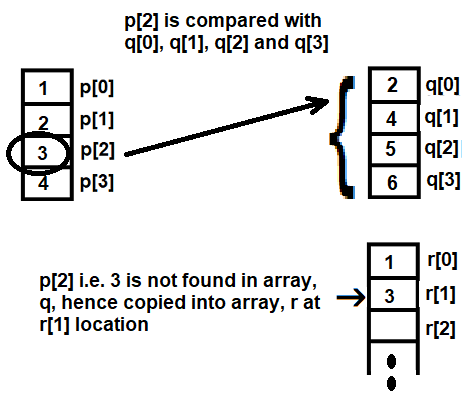Figure 1.16

Continue the procedure until all the elements of array p are compared with all the elements of array q. Finally, we will have array r, with the elements showing the difference between our two arrays, p and q.

Let's use GCC to compile our program, differencearray.c, as follows:

`D:\CBook>gcc differencearray.c -o differencearray`

Now, let's run the generated executable file, differencearray, to see the output of the program:

`D:\CBook>./differencearrayEnter length of first array:4Enter 4 elements of first array1234Enter length of second array:4Enter 4 elements of second array2456The difference of the two array is:13`

Voilà! We've successfully found the difference between two arrays. Now, let's move on to the next recipe!

# Finding the unique elements in an array

In this recipe, we will learn how to find the unique elements in an array, such that the repetitive elements in the array will be displayed only once.

# How to do it…

1. Define two arrays, p and q, of a certain size and assign elements only to array p. We will leave array q blank.
2. These will be our source and target arrays, respectively. The target array will contain the resulting unique elements of the source array.
1. After that, each of the elements in the source array will be compared with the existing elements in the target array.
2. If the element in the source array exists in the target array, then that element is discarded and the next element in the source array is picked up for comparison.
3. If the source array element does not exist in the target array, it is copied into the target array.
4. Let's assume that array p contains the following repetitive elements: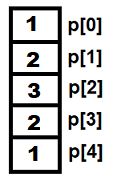Figure 1.17
1. We will start by copying the first element of the source array, p, into the target array, q, in other words, p into array q, as follows: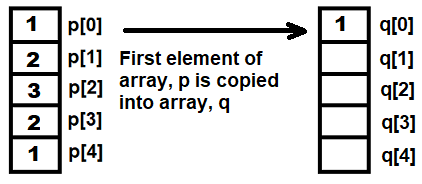Figure 1.18
1. Next, the second array element of p, in other words, p, is compared with all the existing elements of array q. That is, p is compared with array q to check whether it already exists in array q, as follows: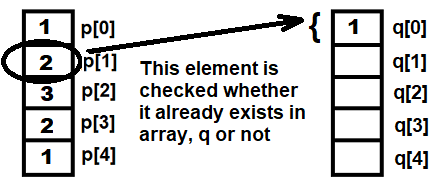Figure 1.19
1. Because p does not exist in array q, it is copied at q, as shown in Figure 1.20: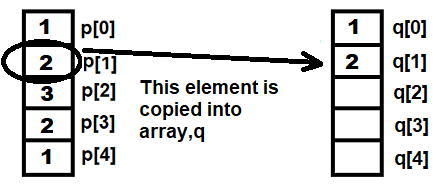Figure 1.20
1. This procedure is repeated until all the elements of array p are compared with array q. In the end, we will have array q, which will contain the unique elements of array p.

Here is the uniqueelements.c program for finding the unique elements in the first array:

`#include<stdio.h>#define max 100int ifexists(int z[], int u, int v){    int i;    for (i=0; i<u;i++)        if (z[i]==v) return (1);    return (0);}void main(){    int p[max], q[max];    int m;    int i,k;    k=0;    printf("Enter length of the array:");    scanf("%d",&m);    printf("Enter %d elements of the array\n",m);    for(i=0;i<m;i++ )        scanf("%d",&p[i]);    q=p;    k=1;    for (i=1;i<m;i++)    {        if(!ifexists(q,k,p[i]))        {            q[k]=p[i];            k++;        }    }    printf("\nThe unique elements in the array are:\n");    for(i = 0;i<k;i++)        printf("%d\n",q[i]);}`

Now, let's go behind the scenes to understand the code better.

# How it works...

We will define a macro called max of size 100. Two arrays, p and q, are defined of size max. Array p will contain the original elements, and array q will contain the unique elements of array p. You will be prompted to enter the length of the array and, thereafter, using the for loop, the elements of the array will be accepted and assigned to array p.

The following statement will assign the first element of array p to the first index location of our blank array, which we will name array q:

`q=p`

A for loop is again used to access the rest of the elements of array p, one by one. First, the foremost element of array p, which is at p, is copied to array q at q.

Next, the second array p element, p, is compared with all the existing elements of array q. That is, p is checked against array q to confirm whether it is already present there.

Because there is only a single element in array q,  p is compared with q. Because p does not exist in array q, it is copied at q.

This procedure is repeated for all elements in array p. Each of the accessed elements of array p is run through the ifexists() function to check whether any of them already exist in array q

The function returns 1 if an element in array p already exists in array q. In that case, the element in array p is discarded and the next array element is picked up for comparison.

In case the ifexists() function returns 0, confirming that the element in array p does not exist in array q, the array p element is added to array q at the next available index/subscript location.

When all the elements of array p are checked and compared, array q will have only the unique elements of array p.

Let's use GCC to compile the uniqueelements.c program as follows:

`D:\CBook>gcc uniqueelements.c -o uniqueelements`

Now, let's run the generated executable file, uniqueelements.exe, to see the output of the program:

`D:\CBook>./uniqueelementsEnter the length of the array:5Enter 5 elements in the array12321The unique elements in the array are:123`

Voilà! We've successfully identified the unique elements in an array. Now, let's move on to the next recipe!

# Finding whether a matrix is sparse

A matrix is considered sparse when it has more zero values than non-zero values (and dense when it has more non-zero values). In this recipe, we will learn how to find out whether the specified matrix is sparse.

# How to do it…

1. First, specify the order of the matrix. Then, you will be prompted to enter the elements in the matrix. Let's assume that you specified the order of the matrix as 4 x 4. After entering the elements in the matrix, it might appear like this: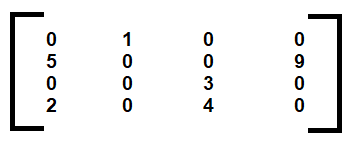Figure 1.21
1. Once the elements of the matrix are entered, count the number of zeros in it. A counter for this purpose is initialized to 0. Using nested loops, each of the matrix elements is scanned and, upon finding any zero elements, the value of the counter is incremented by 1.
1. Thereafter, the following formula is used for establishing whether the matrix is sparse.

If counter > [(the number of rows x the number of columns)/2] =  Sparse Matrix

1. Depending on the result of the preceding formula, one of the following messages will be displayed on the screen as follows:
`The given matrix is a sparse matrix`

or

`The given matrix is not a sparse matrix`

The sparsematrix.c program for establishing whether the matrix is sparse is as follows:

`#include <stdio.h>#define max 100/*A sparse matrix has more zero elements than nonzero elements */void main (){    static int arr[max][max];    int i,j,r,c;    int ctr=0;    printf("How many rows and columns are in this matrix? ");    scanf("%d %d", &r, &c);    printf("Enter the elements in the matrix :\n");    for(i=0;i<r;i++)    {        for(j=0;j<c;j++)        {            scanf("%d",&arr[i][j]);            if (arr[i][j]==0)                ++ctr;        }    }    if (ctr>((r*c)/2))        printf ("The given matrix is a sparse matrix. \n");    else        printf ("The given matrix is not a sparse matrix.\n");    printf ("There are %d number of zeros in the matrix.\n",ctr);}`

Now, let's go behind the scenes to understand the code better.

# How it works...

Because we don't want to fix the size of the matrix, we will define a macro called max of value 100. A matrix, or a two-dimensional array called arr, is defined of the order max x max. You will be prompted to enter the order of the matrix, for which you can again enter any value up to 100.

Let's assume that you’ve specified the order of the matrix as 4 x 4. You will be prompted to enter elements in the matrix. The values entered in the matrix will be in row-major order. After entering the elements, the matrix arr should look like Figure 1.22, as follows: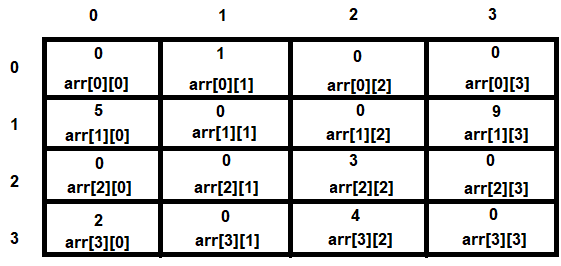Figure 1.22

A counter called ctr is created and is initialized to 0. Using nested loops, each element of matrix arr is checked and the value of ctr is incremented if any element is found to be 0. Thereafter, using the if else statement, we will check whether the count of zero values is more than non-zero values. If the count of zero values is more than non-zero values, then the message will be displayed on the screen as follows:

`The given matrix is a sparse matrix`

However, failing that, the message will be displayed on the screen as follows:

`The given matrix is not a sparse matrix`

Let's use GCC to compile the sparsematrix.c program as follows:

`D:\CBook>gcc sparsematrix.c -o sparsematrix`

Let's run the generated executable file, sparsematrix.exe, to see the output of the program:

`D:\CBook>./sparsematrixHow many rows and columns are in this matrix? 4 4Enter the elements in the matrix :0 1 0 05 0 0 90 0 3 02 0 4 0The given matrix is a sparse matrix.There are 10 zeros in the matrix.`

Okay. Let's run the program again to see the output when the count of non-zero values is higher:

`D:\CBook>./sparsematrixHow many rows and columns are in this matrix? 4 4Enter the elements in the matrix:1 0 3 40 0 2 98 6 5 10 7 0 4The given matrix is not a sparse matrix.There are 5 zeros in the matrix.`

Voilà! We've successfully identified a sparse and a non-sparse matrix.

# There's more...

How about finding an identity matrix, in other words, finding out whether the matrix entered by the user is an identity matrix or not. Let me tell you—a matrix is said to be an identity matrix if it is a square matrix and all the elements of the principal diagonal are ones and all other elements are zeros. An identity matrix of the order 3 x 3 may appear as follows: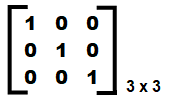Figure 1.23

In the preceding diagram, you can see that the principal diagonal elements of the matrix are 1's and the rest of them are 0's. The index or subscript location of the principal diagonal elements will be arr, arr, and arr, so the following procedure is followed to find out whether the matrix is an identity matrix or not:

• Checks that if the index location of the row and column is the same, in other words, if the row number is 0 and the column number, too, is 0, then at that index location, , the matrix element must be 1. Similarly, if the row number is 1 and the column number, too, is 1, that is, at the  index location, the matrix element must be 1.
• Verify that the matrix element is 0 at all the other index locations.

If both the preceding conditions are met, then the matrix is an identity matrix, or else it is not.

The identitymatrix.c program to establish whether the entered matrix is an identity matrix or not is given as follows:

`    #include <stdio.h>#define max 100/* All the elements of the principal diagonal of the  Identity matrix  are ones and rest all are zero elements  */void main (){    static int arr[max][max];    int i,j,r,c, bool;    printf("How many rows and columns are in this matrix ? ");    scanf("%d %d", &r, &c);    if (r !=c)    {        printf("An identity matrix is a square matrix\n");        printf("Because this matrix is not a square matrix, so it is not an            identity matrix\n");    }    else    {        printf("Enter elements in the matrix :\n");        for(i=0;i<r;i++)        {            for(j=0;j<c;j++)            {                scanf("%d",&arr[i][j]);            }        }        printf("\nThe entered matrix is \n");        for(i=0;i<r;i++)        {            for(j=0;j<c;j++)            {                printf("%d\t",arr[i][j]);            }            printf("\n");        }        bool=1;        for(i=0;i<r;i++)        {            for(j=0;j<c;j++)            {                if(i==j)                {                    if(arr[i][j] !=1)                    {                        bool=0;                        break;                    }                }                else                {                    if(arr[i][j] !=0)                    {                        bool=0;                        break;                    }                }            }        }        if(bool)            printf("\nMatrix is an identity matrix\n");                                     else             printf("\nMatrix is not an identity matrix\n");                    }}`

Let's use GCC to compile the identitymatrix.c program as follows:

`D:\CBook>gcc identitymatrix.c -o identitymatrix`

No error is generated. This means the program is compiled perfectly and an executable file is generated. Let's run the generated executable file. First, we will enter a non-square matrix:

`D:\CBook>./identitymatrixHow many rows and columns are in this matrix ? 3 4An identity matrix is a square matrix Because this matrix is not a square matrix, so it is not an identity matrix`

Now, let's run the program again; this time, we will enter a square matrix

`D:\CBook>./identitymatrix How many rows and columns are in this matrix ? 3 3 Enter elements in the matrix : 1 0 1 1 1 0 0 0 1 The entered matrix is 1       0       1 1       1       0 0       0       1  Matrix is not an identity matrix`

Because a non-diagonal element in the preceding matrix is 1, it is not an identity matrix. Let's run the program again:

`D:\CBook>./identitymatrix How many rows and columns are in this matrix ? 3 3Enter elements in the matrix :1 0 00 1 00 0 1The entered matrix is1       0       00       1       00       0       1Matrix is an identity matrix`

Now, let's move on to the next recipe!

# Merging two sorted arrays into a single array

In this recipe, we will learn to merge two sorted arrays into a single array so that the resulting merged array is also in sorted form.

# How to do it…

1. Let's assume there are two arrays, p and q, of a certain length. The length of the two arrays can differ. Both have some sorted elements in them, as shown in Figure 1.24: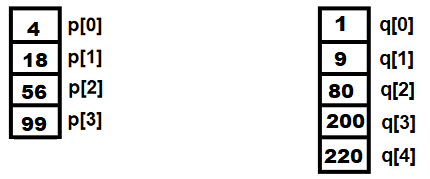Figure 1.24
1. The merged array that will be created from the sorted elements of the preceding two arrays will be called array r. Three subscripts or index locations will be used to point to the respective elements of the three arrays.
2. Subscript i will be used to point to the index location of array p. Subscript j will be used to point to the index location of array q and subscript k will be used to point to the index location of array r. In the beginning, all three subscripts will be initialized to 0.
1. The following three formulas will be applied to get the merged sorted array:
1. The element at p[i] is compared with the element at q[j]. If p[i] is less than q[j], then p[i] is assigned to array r, and the indices of arrays p and r are incremented so that the following element of array p is picked up for the next comparison as follows:
`r[k]=p[i];i++;k++`
1. If q[j] is less than p[i], then q[j] is assigned to array r, and the indices of arrays q and r  are incremented so that the following element of array q is picked up for the next comparison as follows:
`r[k]=q[j];i++;k++`
1. If p[i] is equal to q[j], then both the elements are assigned to array r.  p[i] is added to r[k]. The values of the i and k indices are incremented.  q[j] is also added to r[k], and the indices of the q and r arrays are incremented. Refer to the following code snippet:
`r[k]=p[i];i++;k++r[k]=q[j];i++;k++`
1. The procedure will be repeated until either of the arrays gets over. If any of the arrays is over, the remainder of the elements of the other array will be simply appended to the array r.

The mergetwosortedarrays.c program for merging two sorted arrays is as follows:

`#include<stdio.h>#define max 100void main(){    int p[max], q[max], r[max];    int m,n;    int i,j,k;    printf("Enter length of first array:");    scanf("%d",&m);    printf("Enter %d elements of the first array in sorted order         \n",m);    for(i=0;i<m;i++)        scanf("%d",&p[i]);    printf("\nEnter length of second array:");    scanf("%d",&n);    printf("Enter %d elements of the second array in sorted     order\n",n);    for(i=0;i<n;i++ )        scanf("%d",&q[i]);    i=j=k=0;    while ((i<m) && (j <n))    {        if(p[i] < q[j])        {            r[k]=p[i];            i++;            k++;        }        else        {            if(q[j]< p[i])            {                r[k]=q[j];                k++;                j++;            }            else            {                r[k]=p[i];                k++;                i++;                r[k]=q[j];                k++;                j++;            }        }    }    while(i<m)    {        r[k]=p[i];        k++;        i++;    }    while(j<n)    {        r[k]=q[j];        k++;        j++;    }    printf("\nThe combined sorted array is:\n");    for(i = 0;i<k;i++)        printf("%d\n",r[i]);}`

Now, let's go behind the scenes to understand the code better.

# How it works...

A macro called max is defined of size 100. Three arrays, p, q, and r, are defined of size max. You will first be asked to enter the size of the first array, p, followed by the sorted elements for array p. The process is repeated for the second array q.

Three indices, i, j and k, are defined and initialized to 0. The three indices will point to the elements of the three arrays, p, q, and r, respectively.

The first elements of arrays p and q, in other words, p  and q, are compared and the smaller one is assigned to array r.

Because q is smaller than p, q is added to array rand the indices of arrays q and r are incremented for the next comparison as follows: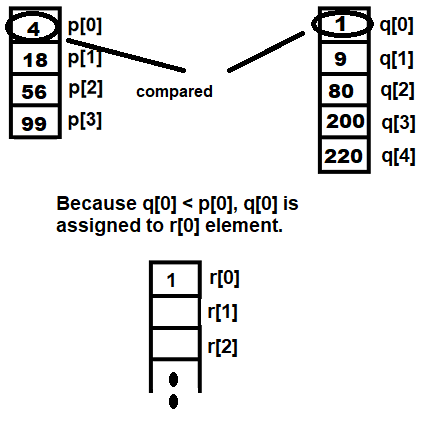Figure 1.25

Next, p will be compared with q. Because p is smaller than q, the value at p will be assigned to array r at r: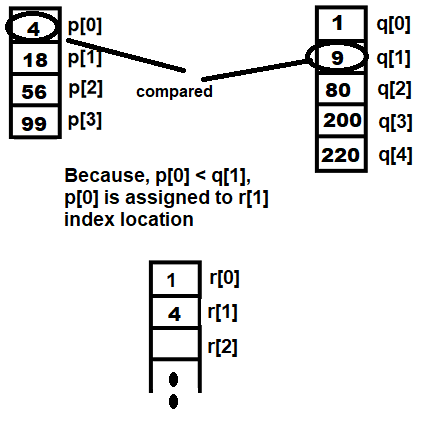Figure 1.26

Then, p will be compared with q. Because q is smaller than p, q will be assigned to array r, and the indices of the q and r arrays will be incremented for the next comparisons (refer to the following diagram):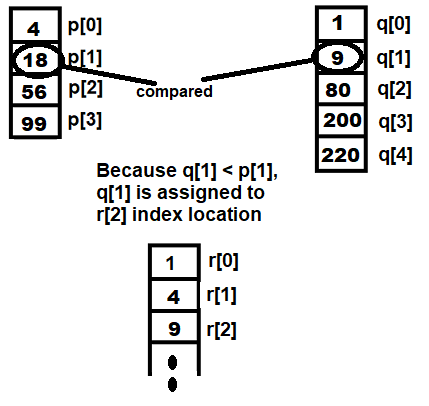Figure 1.27

Let's use GCC to compile the mergetwosortedarrays.c program as follows:

`D:\CBook>gcc mergetwosortedarrays.c -o mergetwosortedarrays`

Now, let's run the generated executable file, mergetwosortedarrays.exe, in order to see the output of the program:

`D:\CBook>./mergetwosortedarraysEnter length of first array:4Enter 4 elements of the first array in sorted order4185699Enter length of second array:5Enter 5 elements of the second array in sorted order1980200220The combined sorted array is:14918568099200220`

Voilà! We've successfully merged two sorted arrays into one.